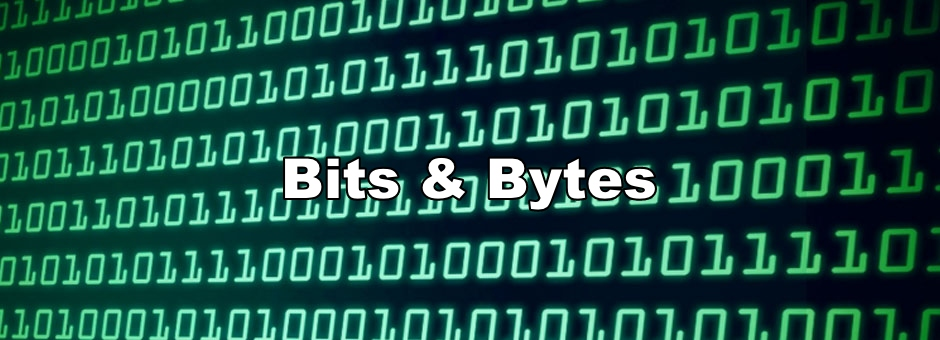Today, we'll be taking a look at a case where addition between two numbers caused a rocket to explode. But first...  A quick review on binary Yesterday, we talked about how computers use binary to internally store information. We saw how we could go from binary to base 10: 10012 = (1 * 23) + (0 * 22) + (0 * 21) + (1 * 20) = 910 We also saw how binary could be used to represent characters and how hexadecimal could be used as shorthand to represent colors. What else can we represent with binary? As we’ve seen before, binary can be used to represent whole numbers. Two of the most common types seen in languages like Java or C are integers (or int for short) or longs. Ints are 4 bytes and longs are 8 bytes long. These types can store 32 bits and 64 bits, respectively. Great! So the range of possible values we can represent spans from 0 to 232 for ints and 0 to 264 for longs, right? But there’s just one problem...  How do we represent negative numbers? It turns out with the current scheme that we have for representing binary numbers, we have no way of indicating negative numbers. One way we could represent negative numbers is having the left most bit indicate whether or not the number is negative, 1 if it is, 0 if it isn’t. But this causes some problems, namely with arithmetic. Consider the following: 410 - 310 = 0000 01002 -  0000 00112 = 0000 00012 = 110 Which works just fine, but: 410 + (-310)= 0000 01002 + 1000 00112 = 1000 01112 = -510 Leads to an incorrect and confusing result. So instead of taking this approach, a different scheme was implemented called Two’s Complement, in which the leftmost bit has a negative weight. For example, the binary number:   1011 10002 Is calculated as: (-1 * 28) + (0 * 27) + (1 * 26) + (1 * 25)  + (1 * 24) + (0 * 23) + (0 * 22) + (0 * 21) + (0 * 20) = -14410 Here’s a useful diagram illustrating the scheme: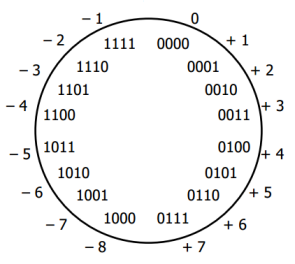A nice trick to go from negative to positive and vice versa is to flip all of the bits of the number, that is, to either go from 0 to 1 or 1 to 0, and add 1. For example, for going from 6 to -6: 610 = 01102 -> 10012 + 00012 = 10102 = -610 In Java, C, and many other languages, both ints and longs are represented using this Two’s Complement scheme. Since this scheme uses 1 bit for a large negative weight, the range for ints and longs is [-231, 231 - 1] and [-263, 263 - 1], respectively. Note that we need to also represent 0 which is why we need to subtract off 1 at the end of the range. Knowing how negative numbers are stored, what implications does this have? Let’s imagine we’re back in the 1990s. We’re just publishing our first website on the internet, Amazon.com, and we want to simulate users trying to hit our website. Well, we could imagine writing a piece of starter code where we simulate people going Amazon.com: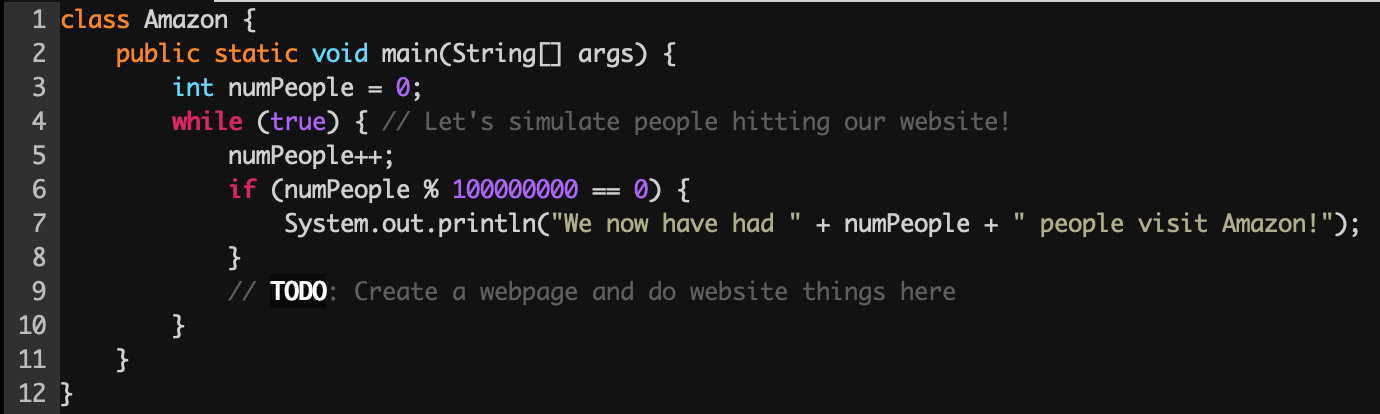Now let’s run the program to see what happens!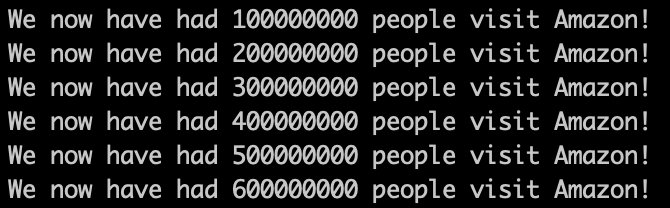Alright, so far so good! But what’s this?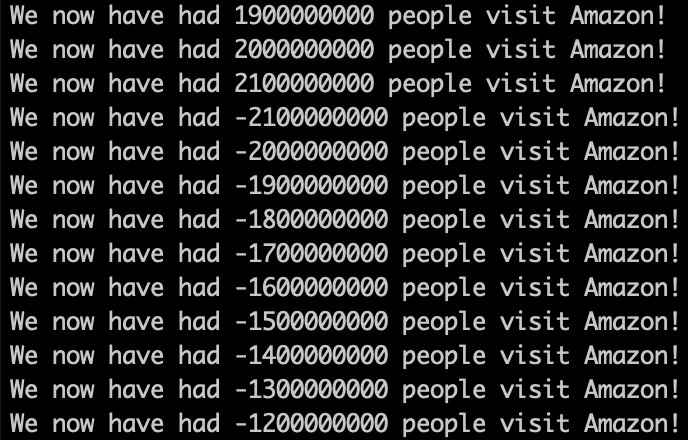Err… how do we now have less than 0 people visiting Amazon? What gives? Remember how integers are represented. They only have so many bits that they can use to represent a number! So when we try adding large numbers, we might get into a situation where we get into negative territory. This phenomenon is referred to as integer overflow. The same is true when subtracting large numbers. We might get in a spot where we are subtracting two negative numbers leading us to wrap back around to a large positive number. This phenomenon is referred to as integer underflow. Here’s another example to illustrate both overflow and underflow: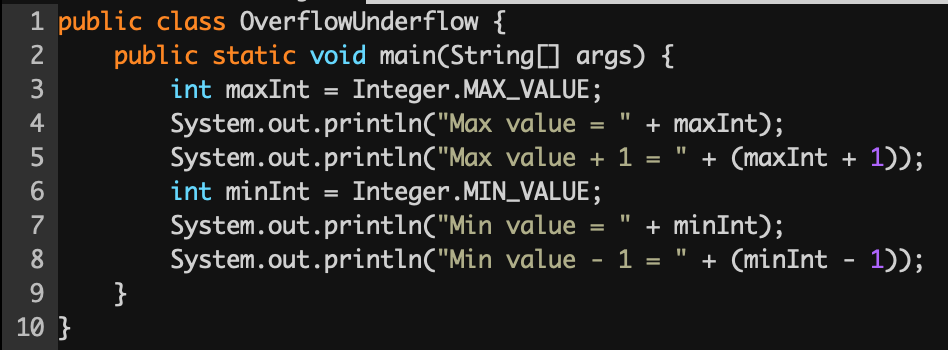And now running the program: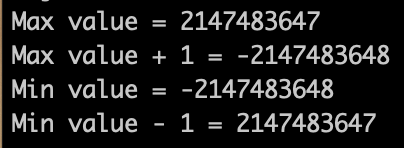This is especially scary because we don’t know when it happens. Our program doesn’t tell us! Implications in real world systems Integer overflow has had a lot of implications on the real world. Cluster, a rocket launched in 1996, exploded when integer overflow caused a hardware exception resulting in 370 million dollars in damages.Another concern resulting from integer overflow is the use of Epoch time. Epoch time is a relative time system that many applications make use of today. It is the number of seconds that have elapsed since Thursday, 1 January 1970. So to represent today’s date, 2/14/2021, we’d represent this with the epoch time of 1613289600.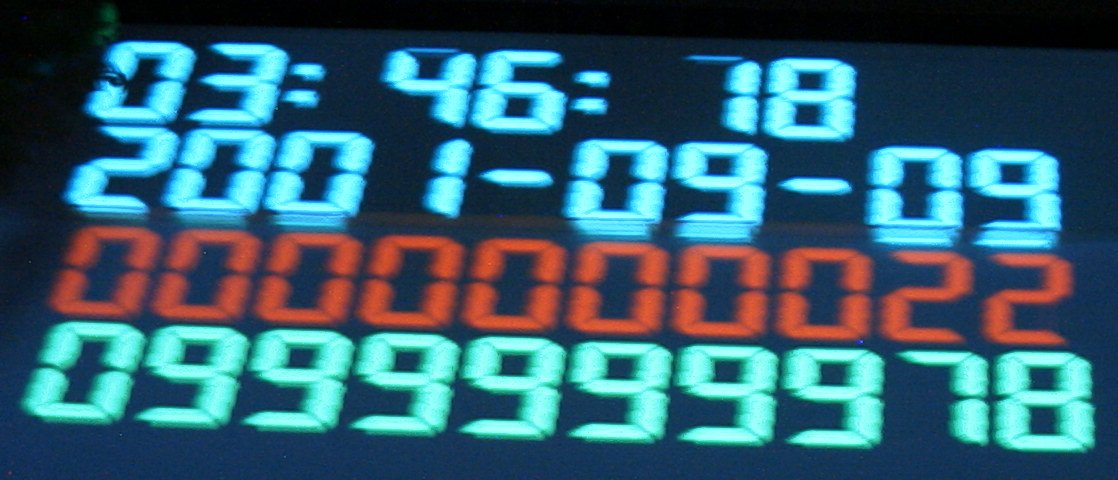Unfortunately, many legacy systems make use of ints, meaning that after January 19, 2038, Epoch will cease the function because of overflow. By that time, 2147483647 (231 - 1) seconds will have passed and epoch time will wrap around back into negative territory. Seeing as the results of overflow & underflow are disastrous, the natural question that follows is, well how do we stop it? Find out in tomorrow’s edition of Beyond the Bootcamp! Feedback I’ve noticed that folks are quite shy about joining the Discord. Not to worry! If you have any feedback, feel free to reply to this email. As always, all previous emails are here in the Discord link if you’d like to join:Justin
 Copyright © 2021 Gestone, All rights reserved. Want to change how you receive these emails? You can update your preferences or unsubscribe from this list.